# Common Source Gate Bias JFET Amplifier Calculator

Inputs:

V
V
mA
V
mA

Theoritical Results: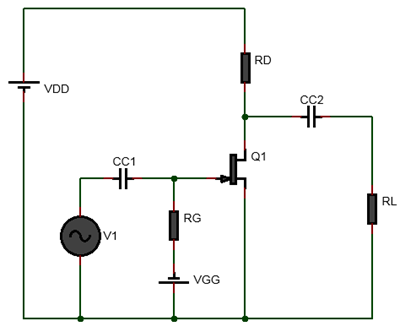### Gate Biased JFET Calculator

The above JFET gate bias calculator helps to calculate the drain current $$I_D$$, drain to source voltage $$V_{DS}$$ from $$I_{DSS}$$, $$V_{GS(off)}$$, $$R_D$$. In gate bias, a negative gate to source voltage is applied to the gate to reverse bias the gate to source junction of the JFET. The gate bias is also known as fixed bias. The gate bias method is usually used to bias the JFET in its ohmic region. It is not suitable for biasing the JFET in its active region due to variation of the JFET parameters. In ohmic region the JFET acts a resistor and a JFET biased with gate bias can be used as variable resistor controlled by the gate to source voltage. The tutorial JFET Gate Bias Circuit with Examples explains step by step the process of biasing a JFET with gate bias(fixed bias).

### Equations Used:

$$\newline$$ (1) $$V_{GS} = V_{GS(off)}(1 - \sqrt{\frac{I_D}{I_{DSS}}})$$ $$\newline$$ (2) $$R_D=\frac{V_{DD}-V_{DS}}{I_D}$$ $$\newline$$ (3) $$Z_i = R_G \space [as \space R_{GS} \space is \space very \space large]$$ $$\newline$$ (4) $$Z_o = R_D \space [assuming \space r_d >> R_D]$$ $$\newline$$ (5) $$CC_1 = \frac{10}{2\pi f Z_i}$$ $$\newline$$ (6) $$CC_2 = \frac{10}{2\pi f Z_o}$$ $$\newline$$

# Common Source JFET Self Bias Amplifier Calculator

Inputs:

V
V
mA

Theoritical Results: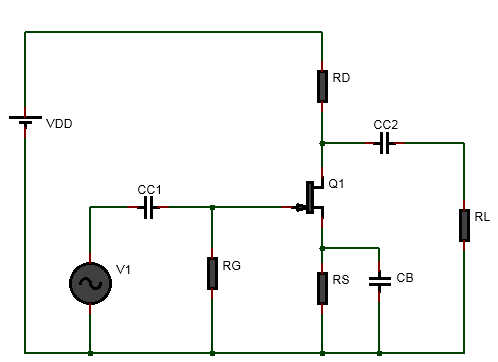### Self Bias JFET Amplifier Design Calculator

This self bias JFET amplifier design calculator helps you to bias a JFET using self biasing method. That is it helps to calculate the drain resistor value and the source resistor value. Furthermore it helps to calculate the coupling capacitors and bypass capacitor values. A detailed worked out example is shown in the tutorial self biased JFET amplifier design.

### Equations Used:

$$\newline$$ (1) $$V_{GS}=\frac{V_{GS(off)}}{3.4}$$$$\newline$$ (2) $$I_D = \frac{I_{DSS}}{2}$$ $$\newline$$ (3) $$R_S = \frac{V_{GS}}{I_D}$$ $$\newline$$ (4) $$R_D=\frac{V_{DD}-V_{D}}{I_{D}}$$,$$\newline$$ (5) $$g_m = \frac{2 I_{DSS}}{V_p} (1-\frac{V_{GS}}{V_p})$$ $$\newline$$ (6) $$R_d = R_D||R_L = \frac{R_DR_L}{R_D+R_L}$$ $$\newline$$ (7) $$A_v = -g_m R_d$$ $$\newline$$ (8) $$Z_i = R_G \space [as \space R_{GS} \space is \space very \space large]$$ $$\newline$$ (9) $$Z_o = R_D \space [Z_o=r_d||R_D\space assuming \space r_d >> R_D]$$ $$\newline$$ (10) $$CC_1 = \frac{10}{2\pi f Z_i}$$ $$\newline$$ (11) $$CC_2 = \frac{10}{2\pi f Z_o}$$ $$\newline$$

# Voltage Divider Biased JFET Amplifier Calculator

Inputs:

V
V
mA
V

Theoritical Results: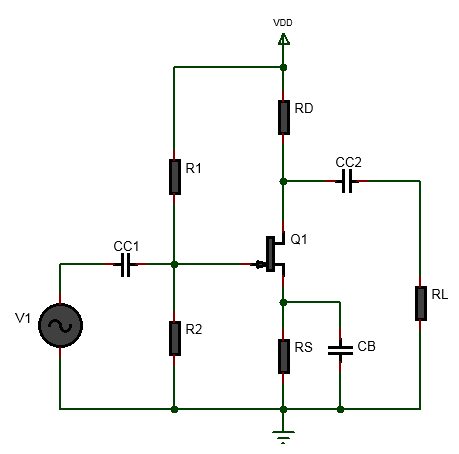### About voltage divider biased JFET amplifier

The JFET voltage divider biasing calculator above calculates the biasing resistor values and coupling and by-pass capacitor values for given input and specification. This is helpful in quick determination for components values for designing JFET amplifiers. Voltage divider biasing is best suited for stable operation among the biasing methods. For detailed steps and explanations see JFET Biasing Worked Out Example Calculation

### Equations Used:

$$\newline$$ (1) $$V_{GS} = \frac{V_{GS(off)}}{2}$$ $$\newline$$ (2) $$V_{G} = -V_{GS(off)}$$ $$\newline$$ (3) $$I_D=\frac{I_{DSS}}{4}$$ $$\newline$$ (4) $$R_{1}=R_2(\frac{V_{DD}}{V_{G}}-1) \space [assume \space R_2]$$ $$\newline$$ (5) $$V_{S}=\frac{V_G-V_{GS}}{I_D}$$ $$\newline$$ (6) $$R_S = \frac{V_S}{I_D}$$ $$\newline$$ (7) $$R_D = \frac{V_{DD}-V_D}{I_D} \space [assume \space V_D]$$ $$\newline$$ (8) $$r_d = R_D || R_L = \frac{R_D R_L}{R_D+R_L}$$ $$\newline$$ (9) $$g_m = \frac{2 I_{DSS}}{V_p} (1-\frac{V_{GS}}{V_p})$$ $$\newline$$ (10) $$A_v = g_m r_d$$ $$\newline$$ (11) $$Z_i = R_1 || R_2 || R_{GS} \approxeq R_1||R_2 \space [as \space R_{GS} \space is \space very \space large]$$ $$\newline$$ (12) $$Z_o = R_D \space \space[Z_o = r_d||R_D \space assuming \space r_d >> R_D]$$ $$\newline$$ (13) $$CC_1 = \frac{10}{2\pi f Z_i}$$ $$\newline$$ (14) $$CC_2 = \frac{10}{2\pi f Z_o}$$ $$\newline$$ (15) $$CB = \frac{10}{2\pi f R_S}$$ $$\newline$$

# Common Drain JFET Amplifier Calculator

Inputs:

V
V
mA

Theoritical Results: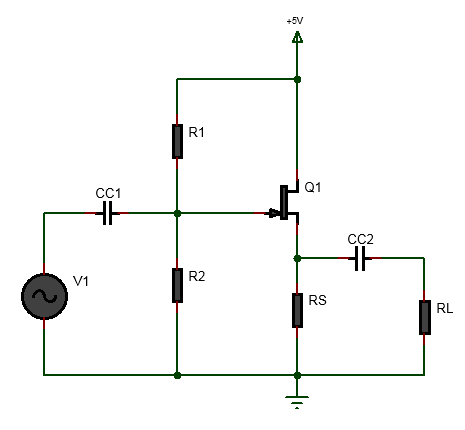The common-drain JFET amplifier is also known as a JFET source follower. In this amplifier, the input is connected to the gate via coupling capacitor and the output is coupled to the load resistor from the source. Such amplifier has a voltage gain less than 1. But there is advantage to using it as it offers very high input resistance and low output resistance. Such common drain JFET amplifier or JFET source follower are often used at the front end of a system which is then followed by BJT amplifiers to provide voltage gain. The tutorial steps to design common drain JFET amplifier illustrates example calculation which uses the equations used in the above JFET common drain amplifier design calculator.

### Equations Used:

$$\newline$$ (1) $$V_{GS} = \frac{V_{GS(off)}}{2}$$ $$\newline$$ (2) $$V_{G} = -V_{GS(off)}$$ $$\newline$$ (3) $$I_D=\frac{I_{DSS}}{4}$$ $$\newline$$ (4) $$R_{1}=R_2(\frac{V_{DD}}{V_{G}}-1) \space [assume \space R_2]$$ $$\newline$$ (5) $$V_{S}=\frac{V_G-V_{GS}}{I_D}$$ $$\newline$$ (6) $$R_S = \frac{V_S}{I_D}$$ $$\newline$$ (7) $$r_s = R_S || R_L = \frac{R_S R_L}{R_S+R_L}$$ $$\newline$$ (8) $$g_m = \frac{2 I_{DSS}}{V_p} (1-\frac{V_{GS}}{V_p})$$ $$\newline$$ (9) $$A_v = \frac{g_m r_s}{1+g_m r_s}$$ $$\newline$$ (10) $$Z_i = R_1 || R_2$$ $$\newline$$ (11) $$Z_o = R_S||\frac{1}{g_m}$$ $$\newline$$ (12) $$CC_1 = \frac{10}{2\pi f Z_i}$$ $$\newline$$ (13) $$CC_2 = \frac{10}{2\pi f Z_o}$$ $$\newline$$

# Common Gate JFET Amplifier Calculator

Inputs:

V
V
mA
V

Theoritical Results: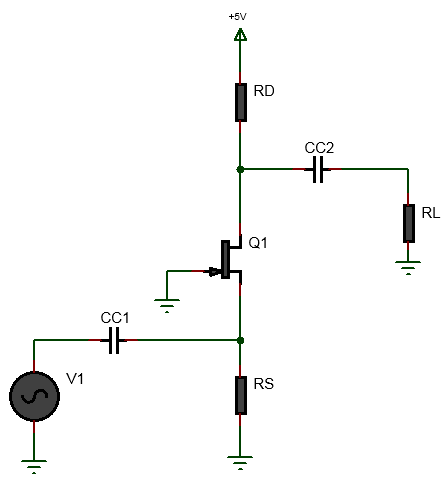## Common Gate JFET amplifier

The common gate JFET(Junction Field-effect Transistors) amplifier is one of the configuration of JFET amplifier. In the common gate JFET amplifier, the gate is grounded, the input is connected to the source via coupling capacitor and the output is coupled to the load resistor from the drain. Such amplifier has a voltage gain greater than 1 but less than 100. It has low input impedance and high output impedance. Common gate JFET are often used as impedance matching circuit and buffer circuit. The tutorial steps to design common drain JFET amplifier illustrates example calculation which uses the equations used in the above JFET common drain amplifier design calculator.

### Equations Used:

$$\newline$$ (1) $$V_{GS} = \frac{V_{GS(off)}}{2}$$ $$\newline$$ (2) $$V_{G} = -V_{GS(off)}$$ $$\newline$$ (3) $$I_D=\frac{I_{DSS}}{4}$$ $$\newline$$ (4) $$V_S=-V_{GS}$$ $$\newline$$ (5) $$V_{DS}= V_D-V_S$$ $$\newline$$ (6) $$R_S = \frac{V_S}{I_D}$$ $$\newline$$ (7) $$r_D = R_D || R_L = \frac{R_D R_L}{R_D+R_L}$$ $$\newline$$ (8) $$g_m = \frac{2 I_{DSS}}{V_p} (1-\frac{V_{GS}}{V_p})$$ $$\newline$$ (9) $$A_v = g_mr_D$$ $$\newline$$ (10) $$Z_i = R_S || \frac{1}{g_m}$$ $$\newline$$ (11) $$Z_o \approxeq R_D$$ $$\newline$$ (12) $$CC_1 = \frac{10}{2\pi f Z_i}$$ $$\newline$$ (13) $$CC_2 = \frac{10}{2\pi f Z_o}$$ $$\newline$$